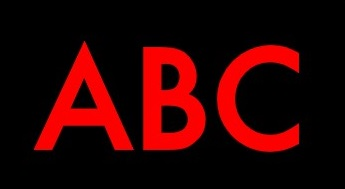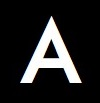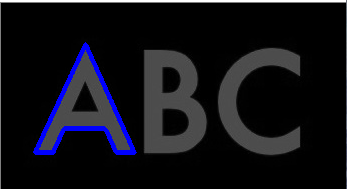# opencv 利用cv.matchShapes()函数实现图像识别技术

## 在待识别图像上找到模板图像## 识别原理

1. 将待识别图像 -> 灰度图像 -> 二值图像
2. 通过轮廓检索函数 cv.findContours 找到待识别图像所有轮廓
3. 模板图像 -> 灰度图像 -> 二值图像
4. 通过轮廓检索函数 cv.findContours 找到模板图像中字母 A 的外轮廓
5. 将第2步得到的轮廓逐一和第4步得到的轮廓 通过 cv.matchShapes 函数进行形状匹配。找到其中最小值，最小值对应的待识别图像中的轮廓即为匹配到的模板图像
6. 标出在待识别图像中找到的模板图像

## 实验：图像匹配

``````import cv2 as cv
import numpy as np

# 载入原图
# 在下面这张图像上作画
image1 = cv.cvtColor(img,cv.COLOR_GRAY2BGR)

# 二值化图像
_, thresh = cv.threshold(img, 0, 255, cv.THRESH_BINARY + cv.THRESH_OTSU)
# 搜索轮廓
contours, hierarchy = cv.findContours(thresh, 3, 2)
hierarchy = np.squeeze(hierarchy)

# 载入标准模板图
_, th = cv.threshold(img_a, 0, 255, cv.THRESH_BINARY + cv.THRESH_OTSU)
contours1, hierarchy1 = cv.findContours(th, 3, 2)
# 字母A的轮廓
template_a = contours1

# 记录最匹配的值的大小和位置
min_pos = -1
min_value = 2
for i in range(len(contours)):
# 参数3：匹配方法；参数4：opencv预留参数
value = cv.matchShapes(template_a,contours[i],1,0.0)
if value < min_value:
min_value = value
min_pos = i

# 参数3为0表示绘制本条轮廓contours[min_pos]
cv.drawContours(image1,[contours[min_pos]],0,[255,0,0],3)

cv.imshow('result',image1)
cv.waitKey(0)
cv.destroyAllWindows()``````

## 实验结果